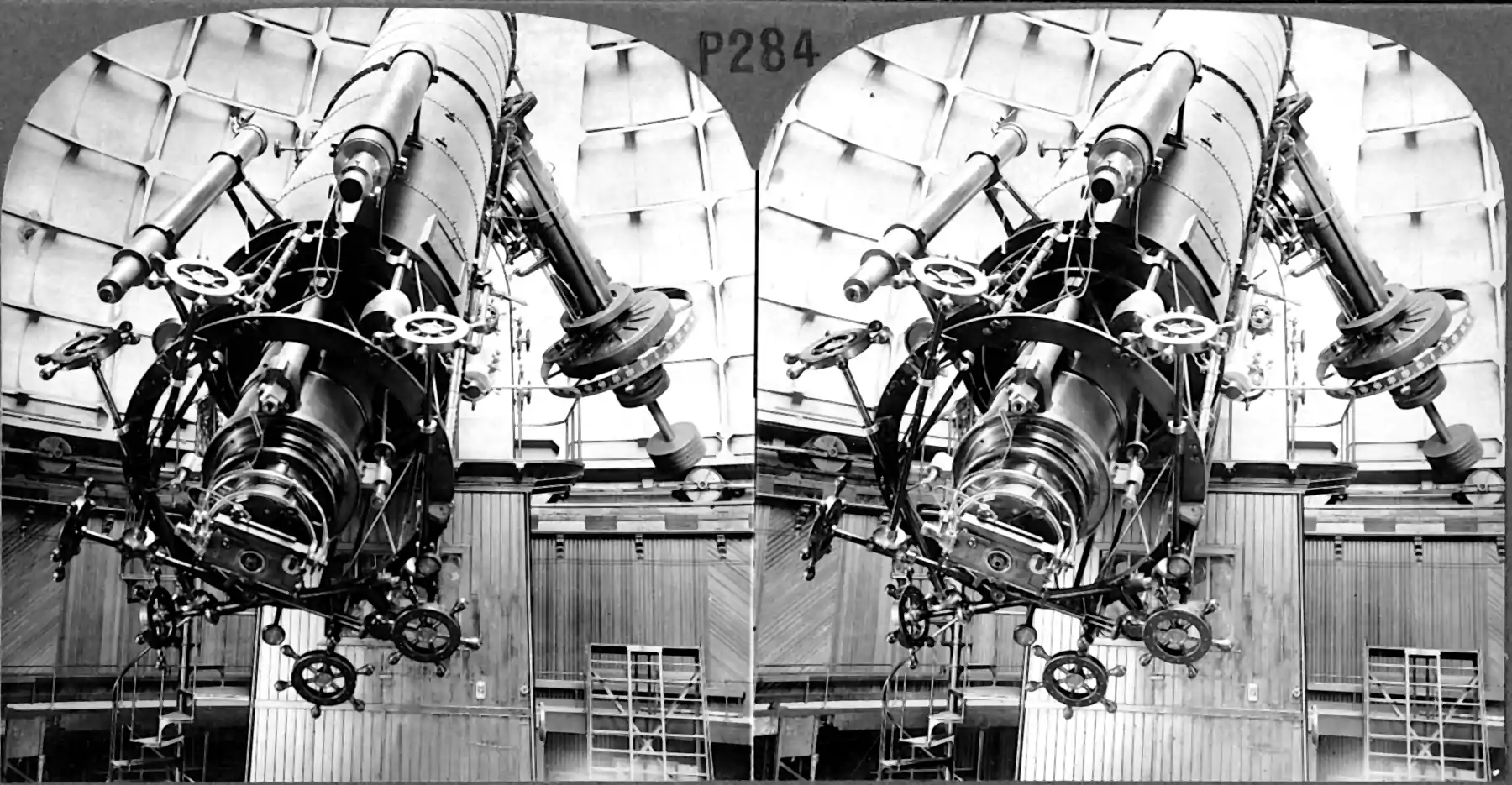# State filtering for hidden Markov models

## Kalman and friendsKalman-Bucy filter and variants, recursive estimation, predictive state models, Data assimilation. A particular sub-field of signal processing for models with hidden state.

In statistics terms, the state filters are a kind of online-updating hierarchical model for sequential observations of a dynamical system where the random state is unobserved, but you can get an optimal estimate of it based on incoming measurements and known parameters.

A unifying feature of all these is by assuming a sparse influence graph between observations and dynamics, that you can estimate behaviour using efficient message passing.

This is a twin problem to optimal control. If I wish to tackle this problem from the perspective of observations rather than true state, perhaps I could do it from the perspective of Koopman operators.

## Linear dynamical systems

In Kalman filters per se the default problem is usually concerned with multivariate real vector signals representing different axes of some telemetry data. In the degenerate case, where there is no observation noise, we can just design a linear filter which solves the target problem.

The classic Kalman filter assumes a linear model with Gaussian noise, although it might work with not-quite Gaussian, not-quite linear models if you prod it. You can extend this flavour to somewhat more general dynamics. For that, see later.

NB I’m conflating linear observation and linear process models, for now. We can relax that when there are some concrete examples in play.

There are a large number of equivalent formulations of the Kalman filter. The notation of Fearnhead and Künsch (2018) is representative. They start from the usual state filter setting: The state process $$\left(\mathbf{X}_{t}\right)$$ is assumed to be Markovian and the $$i$$-th observation, $$\mathbf{Y}_{i}$$, depends only on the state at time $$i, \mathbf{X}_{i}$$, so that the evolution and observation variates are defined by \begin{aligned} \mathbf{X}_{t} \mid\left(\mathbf{x}_{0: t-1}, \mathbf{y}_{1: t-1}\right) & \sim P\left(d \mathbf{x}_{t} \mid \mathbf{x}_{t-1}\right), \quad \mathbf{X}_{0} \sim \pi_{0}\left(d \mathbf{x}_{0}\right) \\ \mathbf{Y}_{t} \mid\left(\mathbf{x}_{0: t}, \mathbf{y}_{1: t-1}\right) & \sim g\left(\mathbf{y}_{t} \mid \mathbf{x}_{t}\right) d \nu\left(\mathbf{y}_{t}\right) \end{aligned} with joint distribution $\left(\mathbf{X}_{0: s}, \mathbf{Y}_{1: t}\right) \sim \pi_{0}\left(d \mathbf{x}_{0}\right) \prod_{i=1}^{s} P\left(d \mathbf{x}_{i} \mid \mathbf{x}_{i-1}\right) \prod_{j=1}^{t} g\left(\mathbf{y}_{j} \mid \mathbf{x}_{j}\right) \nu\left(d \mathbf{y}_{j}\right), \quad s \geq t.$

Integrating out the path of the state process, we obtain that \begin{aligned} \mathbf{Y}_{1: t} &\sim p\left(\mathbf{y}_{1: t}\right) \prod_{j} \nu\left(d \mathbf{y}_{j}\right)\text{, where}\\ p\left(\mathbf{y}_{1: t}\right) &=\int \pi_{0}\left(d \mathbf{x}_{0}\right) \prod_{i=1}^{s} P\left(d \mathbf{x}_{i} \mid \mathbf{x}_{i-1}\right) \prod_{j=1}^{t} g\left(\mathbf{y}_{j} \mid \mathbf{x}_{j}\right). \end{aligned} We wish to find the distribution $$\pi_{0: s \mid t}=\frac{p(\mathbf{y}_{1: t},\mathbf{x}_{0:s})}{p(\mathbf{y}_{1: t})}$$ (by Bayes’ rule). We deduce the recursion \begin{aligned} \pi_{0: t \mid t-1}\left(d \mathbf{x}_{0: t} \mid \mathbf{y}_{1: t-1}\right) &=\pi_{0: t-1 \mid t-1}\left(d \mathbf{x}_{0: t-1} \mid \mathbf{y}_{1: t-1}\right) P\left(d \mathbf{x}_{t} \mid \mathbf{x}_{t-1}\right) &\text{ prediction}\\ \pi_{0: t \mid t}\left(d \mathbf{x}_{0: t} \mid \mathbf{y}_{1: t}\right) &=\pi_{0: t \mid t-1}\left(d \mathbf{x}_{0: t} \mid \mathbf{y}_{1: t-1}\right) \frac{g\left(\mathbf{y}_{t} \mid \mathbf{x}_{t}\right)}{p\left(\mathbf{y}_{t} \mid \mathbf{y}_{1: t-1}\right)} &\text{ correction} \end{aligned} where $p\left(\mathbf{y}_{t} \mid \mathbf{y}_{1: t-1}\right)=\frac{p\left(\mathbf{y}_{1: t}\right)}{p\left(\mathbf{y}_{1: t-1}\right)}=\int \pi_{t \mid t-1}\left(d \mathbf{x}_{t} \mid \mathbf{y}_{1: t-1}\right) g\left(\mathbf{y}_{t} \mid \mathbf{x}_{t}\right) .$ Integrating out all but the latest states $$\mathbf{x}_{0: t-1}$$ gives us the one-step recursion \begin{aligned} \pi_{t \mid t-1}\left(d \mathbf{x}_{t} \mid \mathbf{y}_{1: t-1}\right) &=\int \pi_{t-1}\left(d \mathbf{x}_{t-1} \mid \mathbf{y}_{1: t-1}\right) P\left(d \mathbf{x}_{t} \mid \mathbf{x}_{t-1}\right) &\text{ prediction}\\ \pi_{t}\left(d \mathbf{x}_{t} \mid \mathbf{y}_{1: t}\right) &=\pi_{t \mid t-1}\left(d \mathbf{x}_{t} \mid \mathbf{y}_{1: t-1}\right) \frac{g\left(\mathbf{y}_{t} \mid \mathbf{x}_{t}\right)}{p_{t}\left(\mathbf{y}_{t} \mid \mathbf{y}_{1: t-1}\right)}&\text{ correction} \end{aligned}

If we approximate the filter distribution $$\pi_t$$ with a Monte Carlo sample, we are doing particle filtering, which Fearnhead and Künsch (2018) refer to as bootstrap filtering.

TODO: implied Kalman gain etc.

## Non-linear dynamical systems

Cute exercise: you can derive the analytic Kalman filter for any noise and process dynamics of with Bayesian conjugate, and this leads to filters of nonlinear behaviour. Multivariate distributions are a bit of a mess for non-Gaussians, though, and a beta-Kalman filter feels contrived.

Upshot is, the non-linear extensions don’t usually rely on non-Gaussian conjugate distributions and analytic forms, but rather do some Gaussian/linear approximation, or use randomised methods such as particle filters.

For some examples in Stan see Sinhrks’ stan-statespace.

## As errors-in-variables models

see, e.g. Bagge Carlson (2018).

## Discrete state Hidden Markov models

🏗 Viterbi algorithm.

## Unscented Kalman filter

i.e. using the unscented transform.

## State filter inference

How about learning the parameters of the model generating your states? Ways that you can do this in dynamical systems include basic linear system identification, general system identification, .

### No comments yet. Why not leave one?

GitHub-flavored Markdown & a sane subset of HTML is supported.